# Luzin-Privalov theorems

in the theory of functions of a complex variable

Classical results of N.N. Luzin and I.I. Privalov that clarify the character of a boundary uniqueness property of analytic functions (cf. Uniqueness properties of analytic functions) (see ).

1) Letbe a meromorphic function of the complex variablein a simply-connected domainwith rectifiable boundary. Iftakes angular boundary values zero on a setof positive Lebesgue measure on, thenin. There is no function meromorphic inthat has infinite angular boundary values on a set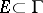of positive measure.

2) Let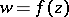be a meromorphic function in the unit disc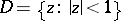other than a constant and having radial boundary values (finite or infinite) on a setsituated on an arcof the unit circle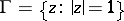that is metrically dense and of the second Baire category (cf. Baire classes) on. Then the setof its radial boundary values oncontains at least two distinct points. Metric density ofonmeans that every portion ofonhas positive measure. This implies that if the radial boundary values ofon a setof the given type are equal to zero, then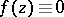in. Moreover, there is no meromorphic function in the unit disc that takes infinite radial boundary values on a setof the given type.

Luzin and Privalov (see , ) constructed examples to show that neither metric density nor second Baire category are by themselves sufficient for the assertion in 2 to hold.

How to Cite This Entry:
Luzin-Privalov theorems. Encyclopedia of Mathematics. URL: http://encyclopediaofmath.org/index.php?title=Luzin-Privalov_theorems&oldid=12447
This article was adapted from an original article by E.D. Solomentsev (originator), which appeared in Encyclopedia of Mathematics - ISBN 1402006098. See original article